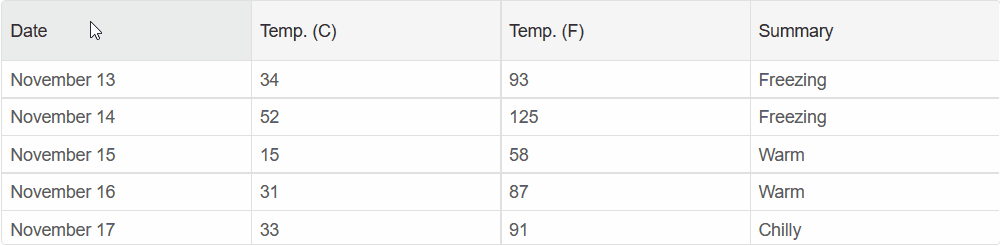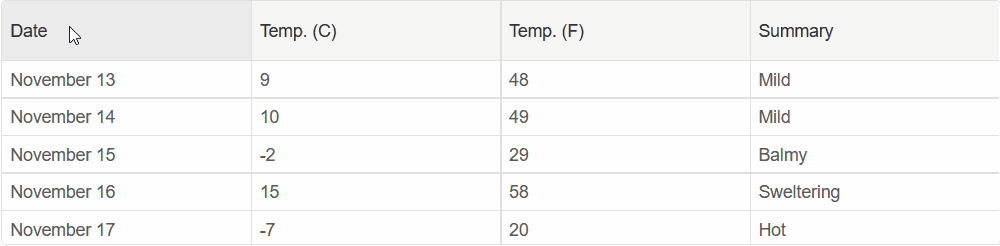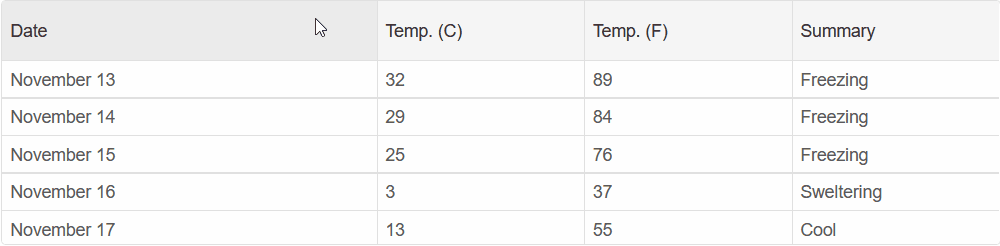Blazor Grid - Column Resize

## Resize Columns

Smart.Grid allows you to customize which columns can have their resizing enabled. To enable resizing set the column's `AllowResize` property to true:

```@inject WeatherForecastService ForecastService

<Grid DataSource="@forecast" >
<Columns>
<Column DataField="Date" Label="Date" AllowResize="true"> </Column>
<Column DataField="TemperatureC" Label="Temp. (C)" AllowResize="true"> </Column>
<Column DataField="TemperatureF" Label="Temp. (F)" AllowResize="true"> </Column>
<Column DataField="Summary" Label="Summary" AllowResize="true"> </Column>
</Columns>
</Grid>

@code{
private WeatherForecast[] forecast;

{
forecast = await ForecastService.GetForecastAsync(DateTime.Now);
}
}```

Then you need to select the ResizeMode of the Grid using the GridBehavior object. The `ColumnResizeMode` can be set to either Split or GrowAndShrink

```<Grid DataSource="@forecast" Behavior="gridBehavior">
....
</Grid>

@code{
GridBehavior gridBehavior = new GridBehavior(){
ColumnResizeMode = GridResizeMode.Split
};
....
}```

When `GridResizeMode` is set to Split, the expanding column will take up space from the next sibling column:When `GridResizeMode` is set to GrowAndShrink, the expanding column will take up space by shrinking all of the subsequent columns:### Column Width

Using the column's `Width` and `MinWidth` properties, you can set the size of the Column. When resizing, the column will not shrink below the value set in `MinWidth`

```<Grid DataSource="@forecast" Behavior="gridBehavior">
<Columns>
<Column DataField="Date" Label="Date" AllowResize="true" MinWidth="150" Width="300"> </Column>
<Column DataField="TemperatureC" Label="Temp. (C)" AllowResize="true" MinWidth="80"> </Column>
<Column DataField="TemperatureF" Label="Temp. (F)" AllowResize="true" MinWidth="80"> </Column>
<Column DataField="Summary" Label="Summary" AllowResize="true" MinWidth="100"> </Column>
</Columns>
</Grid>
```